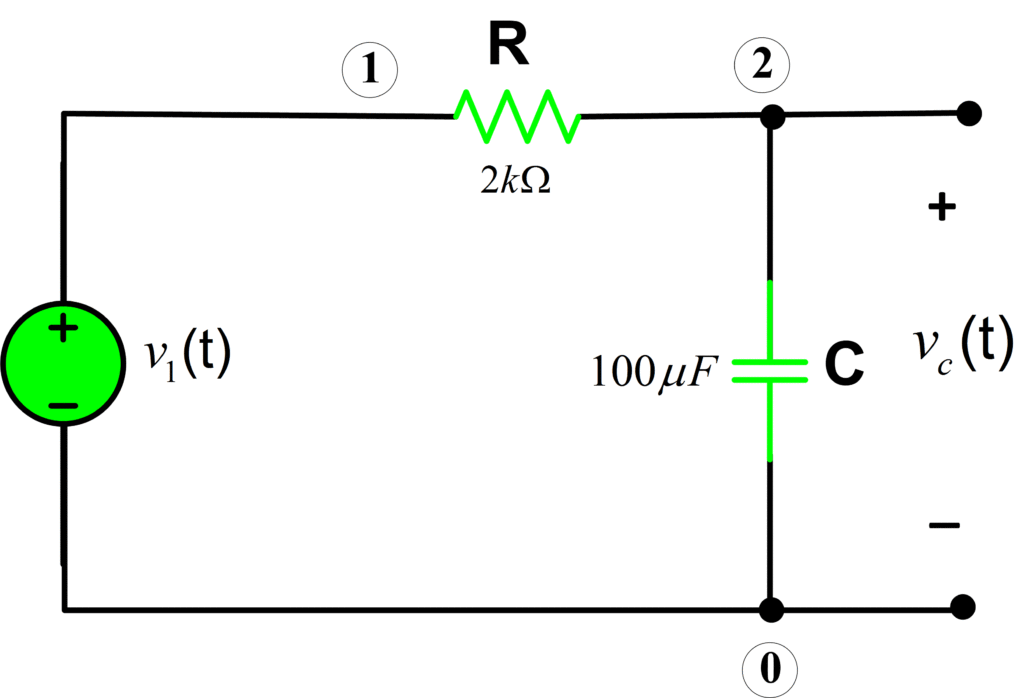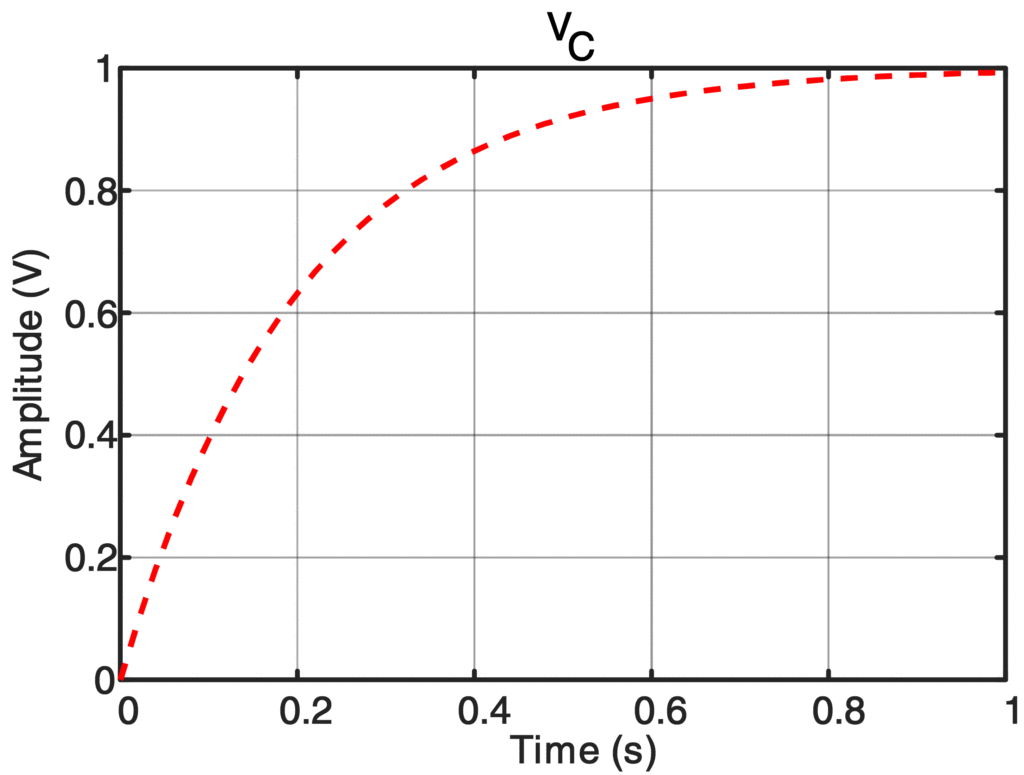Home / Circuits with Matlab / Capacitor Charging Equation | RC Circuit Charging | Matlab

# Capacitor Charging Equation | RC Circuit Charging | Matlab

Want create site? Find Free WordPress Themes and plugins.

In this tutorial, we will Calculate Voltage Across the Capacitor in RC Circuit Using Matlab.RC circuit charging expression is also discussed.

Determine the voltage across the capacitor:Let us compute the voltage across the capacitor for t≥0 using the following expression:

${{v}_{C}}(t)={{V}_{s}}(1-{{e}^{-t/\tau }})u(t)$

Whereas the source voltage is 1V and time constant τ=RC=0.2s.

It’s time to write some code in Matlab to calculate the capacitor voltage:

%RC Circuit Analysis with Source Added
clear all;close all;clc
%%Circuit Parameters
R= 2e3; % Resistance (2kOhm)
C= 100e-6; % Capacitance (100microFarad)
tau=R*C; % Circuit Time Constant
Vs= 1; % Source Voltage
Time= 0:tau/10:5*tau; % Sampling Time
V_C= Vs.*(1-exp(-Time./tau)).*heaviside(Time);
%%Plotting the Result
plot(Time,V_C)
xlabel('Time (s)')
ylabel('Amplitude (V)')
title('V_C')
%=============================================


Results:Did you find apk for android? You can find new Free Android Games and apps.